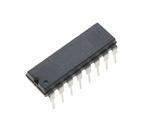< id="6e2kg">< id="6e2kg">< id="6e2kg">< id="6e2kg">< id="6e2kg">< id="6e2kg">< id="6e2kg">< id="6e2kg">< id="6e2kg">< id="6e2kg">< id="6e2kg">< id="6e2kg">< id="6e2kg">< id="6e2kg">< id="6e2kg">< id="6e2kg">< id="6e2kg">< id="6e2kg">< id="6e2kg">< id="6e2kg">< id="6e2kg">< id="6e2kg">< id="6e2kg">< id="6e2kg">< id="6e2kg">< id="6e2kg">< id="6e2kg">< id="6e2kg">< id="6e2kg">< id="6e2kg">< id="6e2kg">< id="6e2kg">< id="6e2kg">< id="6e2kg">< id="6e2kg">< id="6e2kg">< id="6e2kg">< id="6e2kg">< id="6e2kg">< id="6e2kg">< id="6e2kg">< id="6e2kg">< id="6e2kg">< id="6e2kg">< id="6e2kg">< id="6e2kg">< id="6e2kg">< id="6e2kg">< id="6e2kg">< id="6e2kg"> < id="6e2kg">< id="6e2kg">< id="6e2kg">< id="6e2kg">< id="6e2kg">< id="6e2kg">< id="6e2kg">< id="6e2kg">< id="6e2kg">< id="6e2kg">< id="6e2kg">< id="6e2kg">< id="6e2kg">< id="6e2kg">< id="6e2kg">< id="6e2kg">< id="6e2kg">< id="6e2kg">< id="6e2kg">< id="6e2kg">< id="6e2kg">< id="6e2kg">< id="6e2kg">< id="6e2kg">< id="6e2kg">< id="6e2kg">< id="6e2kg">< id="6e2kg">< id="6e2kg">< id="6e2kg">< id="6e2kg">< id="6e2kg">< id="6e2kg">< id="6e2kg">< id="6e2kg">< id="6e2kg">< id="6e2kg">< id="6e2kg">< id="6e2kg">< id="6e2kg">< id="6e2kg">< id="6e2kg">< id="6e2kg">< id="6e2kg">< id="6e2kg">< id="6e2kg">< id="6e2kg">< id="6e2kg">< id="6e2kg">< id="6e2kg"> < id="6e2kg">< id="6e2kg">< id="6e2kg">< id="6e2kg">< id="6e2kg">< id="6e2kg">< id="6e2kg">< id="6e2kg">< id="6e2kg">< id="6e2kg">< id="6e2kg">< id="6e2kg">< id="6e2kg">< id="6e2kg">< id="6e2kg">< id="6e2kg">< id="6e2kg">< id="6e2kg">< id="6e2kg">< id="6e2kg">< id="6e2kg">< id="6e2kg">< id="6e2kg">< id="6e2kg">< id="6e2kg">< id="6e2kg">< id="6e2kg">< id="6e2kg">< id="6e2kg">< id="6e2kg">< id="6e2kg">< id="6e2kg">< id="6e2kg">< id="6e2kg">< id="6e2kg">< id="6e2kg">< id="6e2kg">< id="6e2kg">< id="6e2kg">< id="6e2kg">< id="6e2kg">< id="6e2kg">< id="6e2kg">< id="6e2kg">< id="6e2kg">< id="6e2kg">< id="6e2kg">< id="6e2kg">< id="6e2kg">< id="6e2kg">型号： ULN2003A 厂商：STMicroelectronics 标准：分类： 半导体 ， 分离式半导体 描述： transistors darlington seven npn array PDF： 预览 报错 收藏 赞

Datasheet下载地址

 本地下载 >> 厂商下载 >> 厂商下载2 >>

ULN2003A的详细信息

Manufacturer： STMicroelectronics Transistors Darlington Yes STMicroelectronics Array 7 NPN 50 V 0.5 A + 85 C Through Hole PDIP-16 Tube - 20 C ULN2003 25
ULN2001, ULN2002
ULN2003, ULN2004
Seven Darlington array
Datasheet ? production data
Features
Seven Darlingtons per package
Output current 500 mA per driver (600 mA
peak)
Output voltage 50 V
Integrated suppression diodes for inductive
Outputs can be paralleled for higher current
TTL/CMOS/PMOS/DTL compatible inputs
DIP-16
SO-16
(Narrow)
Inputs pinned opposite outputs to simplify
layout
These versatile devices are useful for driving a
wide range of loads including solenoids, relays
DC motors, LED displays filament lamps, thermal
printheads and high power buffers.
Description
The ULN2001, ULN2002, ULN2003 and ULN
2004 are high voltage, high current Darlington
arrays each containing seven open collector
Darlington pairs with common emitters. Each
channel rated at 500 mA and can withstand peak
currents of 600 mA. Suppression diodes are
included for inductive load driving and the inputs
are pinned opposite the outputs to simplify board
layout.
The ULN2001A/2002A/2003A and 2004A are
supplied in 16 pin plastic DIP packages with a
copper leadframe to reduce thermal resistance.
They are available also in small outline package
(SO-16) as ULN2001D1/2002D1/2003D1/
2004D1
The versions interface to all common logic
families:
– ULN2001 (general purpose, DTL, TTL,
PMOS, CMOS)
– ULN2002 (14 - 25 V PMOS)
– ULN2003 (5 V TTL, CMOS)
– ULN2004 (6 - 15 V CMOS, PMOS)
Table 1.
Device summary
Order codes
ULN2001A
ULN2002A
ULN2003A
ULN2004A
ULN2001D1013TR
ULN2002D1013TR
ULN2003D1013TR
ULN2004D1013TR
June 2012
Doc ID 5279 Rev 8
1/16
This is information on a product in full production.
16